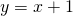# Solution assignment 10 Tangent line to graph

### Assignment 10

Give the equation of the line through the points:and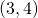### Solution

The slope of the line through the points: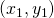and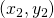can be calculated as follows: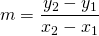For the given points this yields: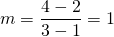Now we also know the slope of the line and thus we can use the following formula for the line: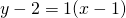or: